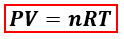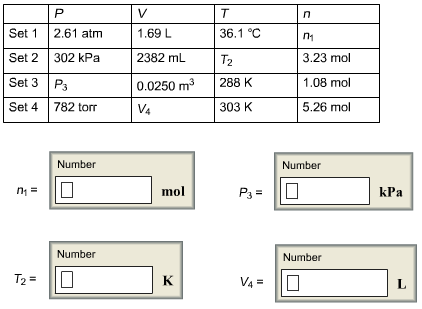# Problem: For each set of values, calculate the missing variable using the ideal gas law.

###### FREE Expert Solution

We will use the ideal gas equation to calculate for each of theP = pressure, atm
V = volume, L
n = moles, mol
R = gas constant = 0.08206 (L·atm)/(mol·K)
T = temperature, K

It is important to note the units used for this formula. We can see that our given does not have the same units so we would need to convert.

84% (316 ratings)###### Problem Details

For each set of values, calculate the missing variable using the ideal gas law.